﻿ 顾及OSB改正的BDS-3新频点精密单点定位精度分析文章快速检索 高级检索
 大地测量与地球动力学2023, Vol. 43Issue (1): 18-22  DOI: 10.14075/j.jgg.2023.01.004### 引用本文GU Jiachen, SONG Chuanfeng, TIAN Kunjun. Precision Single Point Positioning Accuracy Analysis of BDS-3 New Frequencies Based on OSB Correction[J]. Journal of Geodesy and Geodynamics, 2023, 43(1): 18-22.### Corresponding author

SONG Chuanfeng, PhD candidate, majors in Beidou/GNSS precise single point positioning and deviation estimation, E-mail: song_chuan_feng@whu.edu.cn.

### 第一作者简介

GU Jiachen, engineer, majors in GNSS high precision data processing, E-mail: gu806142978@163.com.

### 文章历史

1. 山东无形信息技术有限公司，山东省泰安市御碑楼路67号，271000;
2. 武汉大学卫星导航定位技术研究中心，武汉市珞喻路129号，430079;
3. 山东理工大学建筑工程学院，山东省淄博市新村西路266号，255000

BDS-2/3采用双频伪距观测量和双频载波相位观测量进行伪距单点定位(SPP)和精密单点定位(PPP)，其理论方法和实现算法已经成熟[1-3]。然而，目前最常用的双频消电离层组合(IF)方法虽然可以消除一阶项电离层误差，但在解算过程中会出现卫星端与接收机端间的硬件延迟，且地面接收机在接收信号时也会存在由天线和仪器设备等造成的测距码偏差，上述偏差统称为观测量码偏差[5-6]。观测量码偏差一直是精密定位研究中的热点，通常将差分定位解算中的观测量偏差称为差分码偏差DSB或DCB，将IF组合定位解算中的观测量偏差称为ISB[7-8]，国内外学者在观测量码偏差方面做了大量研究[9-14]

1 OSB估计模型与稳定性分析 1.1 OSB估计模型

 $\left\{\begin{array}{c} P_{\mathrm{r}, x}^{\mathrm{s}}=\rho_{\mathrm{r}}^{\mathrm{s}}+I_{\mathrm{r}, x}^{\mathrm{s}}+T_{\mathrm{r}}^{\mathrm{s}}+c \cdot \\ \quad\left(\mathrm{d} t_{\mathrm{r}}-\mathrm{d} t^{\mathrm{s}}\right)+B_{\mathrm{r}, x}+B_x^{\mathrm{s}} \\ P_{\mathrm{r}, y}^{\mathrm{s}}=\rho_{\mathrm{r}}^{\mathrm{s}}+I_{\mathrm{r}, y}^{\mathrm{s}}+T_{\mathrm{r}}^{\mathrm{s}}+c \cdot \\ \quad\left(\mathrm{d} t_{\mathrm{r}}-\mathrm{d} t^{\mathrm{s}}\right)+B_{\mathrm{r}, y}+B_y^{\mathrm{s}} \end{array}\right.$ (1)

 $\begin{gathered} P_{\mathrm{r}, \mathrm{GF}}^{\mathrm{s}}=P_{\mathrm{r}, x}^{\mathrm{s}}-P_{\mathrm{r}, y}^{\mathrm{s}}=40.3\left(\frac{1}{f_x^2}-\frac{1}{f_y^2}\right) \cdot \\ \mathrm{STEC}_{\mathrm{r}}^{\mathrm{s}}+B_{r, x}-B_{\mathrm{r}, y}+B_x^{\mathrm{s}}-B_y^{\mathrm{s}} \end{gathered}$ (2)

 $\begin{gathered} \mathrm{DCB}_{\mathrm{r}, x y}^{\mathrm{s}}=\mathrm{DCB}_{\mathrm{r}, x y}+\mathrm{DCB}_{x y}^{\mathrm{s}}= \\ B_{\mathrm{r}, x}-B_{\mathrm{r}, y}+B_x^s-B_y^s \end{gathered}$ (3)

 $\frac{1}{N_x} \sum\limits_{i=1}^{N_x} B_x^{\mathrm{s}}=0$ (4)

 $\left\{\begin{array}{l} \frac{f_x^2}{f_x^2-f_y^2} B_x^{\mathrm{s}}-\frac{f_y^2}{f_x^2-f_y^2} B_y^{\mathrm{s}}=0 \\ \frac{f_x^2}{f_x^2-f_y^2} B_{\mathrm{r}, x}-\frac{f_y^2}{f_x^2-f_y^2} B_{\mathrm{r}, y}=0 \end{array}\right.$ (5)

1.2 BDS-2/3卫星OSB稳定性分析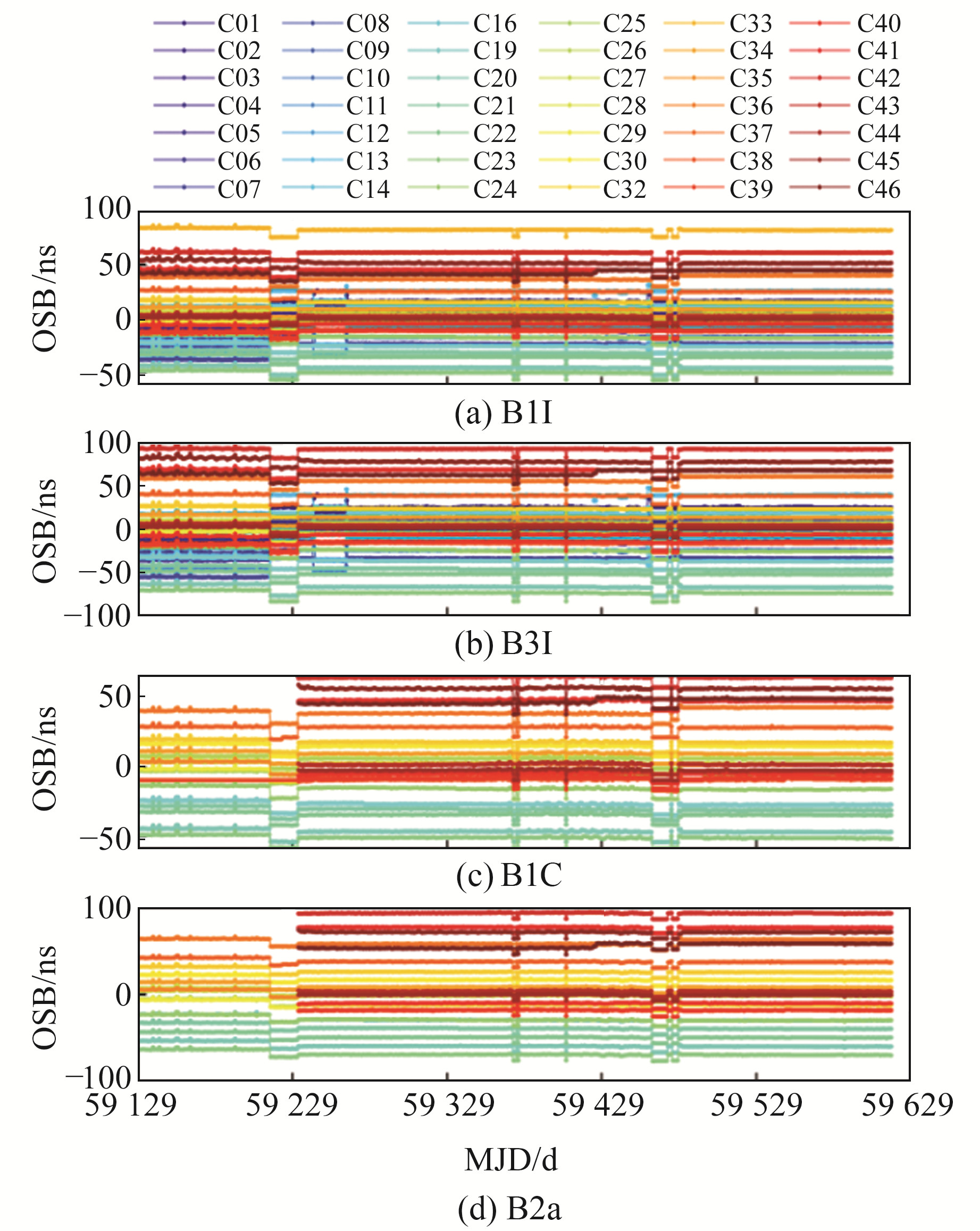图 1 各频点OSB值时间序列 Fig. 1 Time series of OSB values at each frequency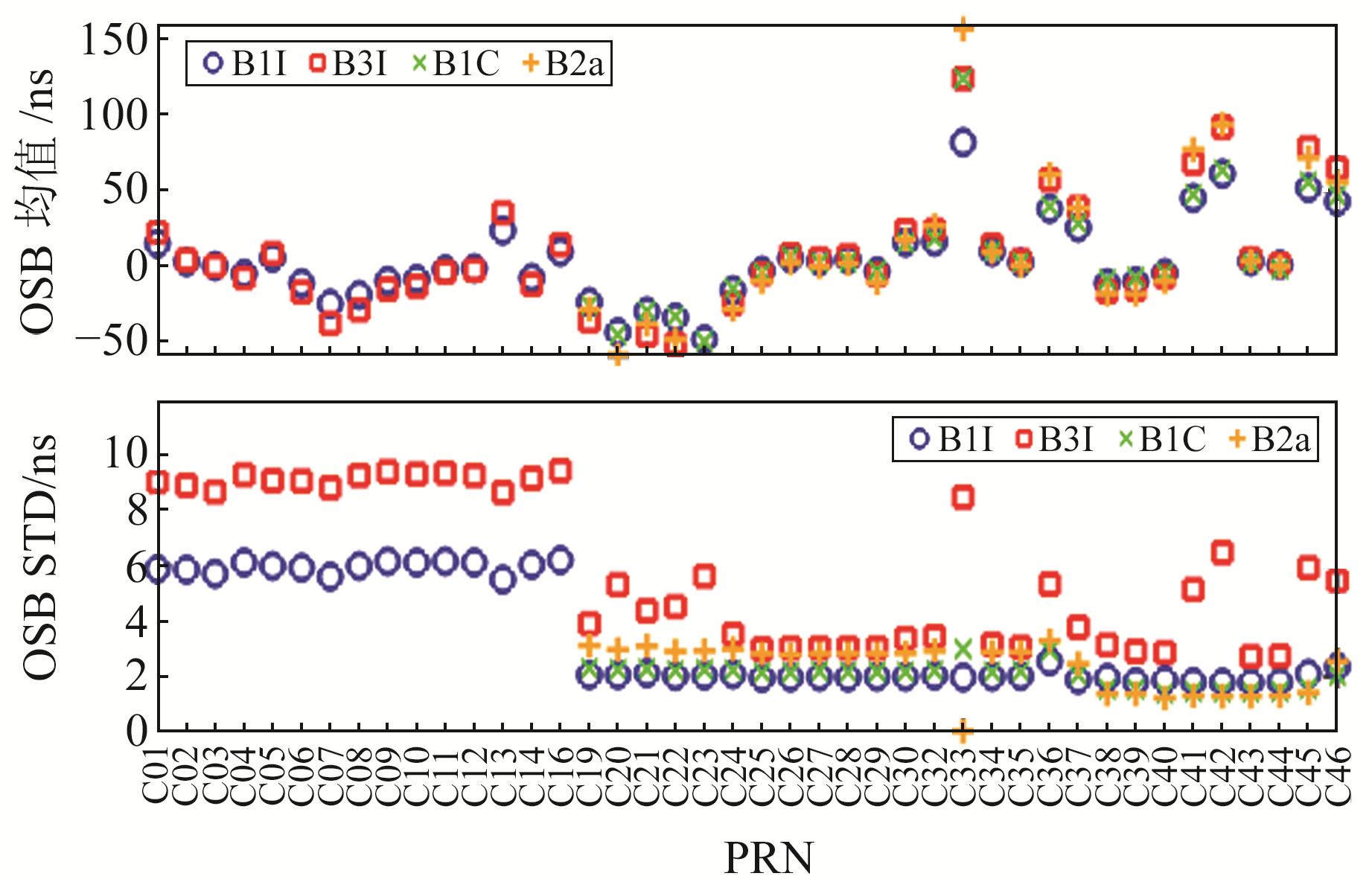图 2 BDS-2/3各卫星各频点OSB均值和STD值 Fig. 2 OSB mean value and STD value of each satellite at each frequency point of BDS-2/3
2 OSB改正算例分析表 1 精密定位处理策略 Tab. 1 Precision positioning processing strategy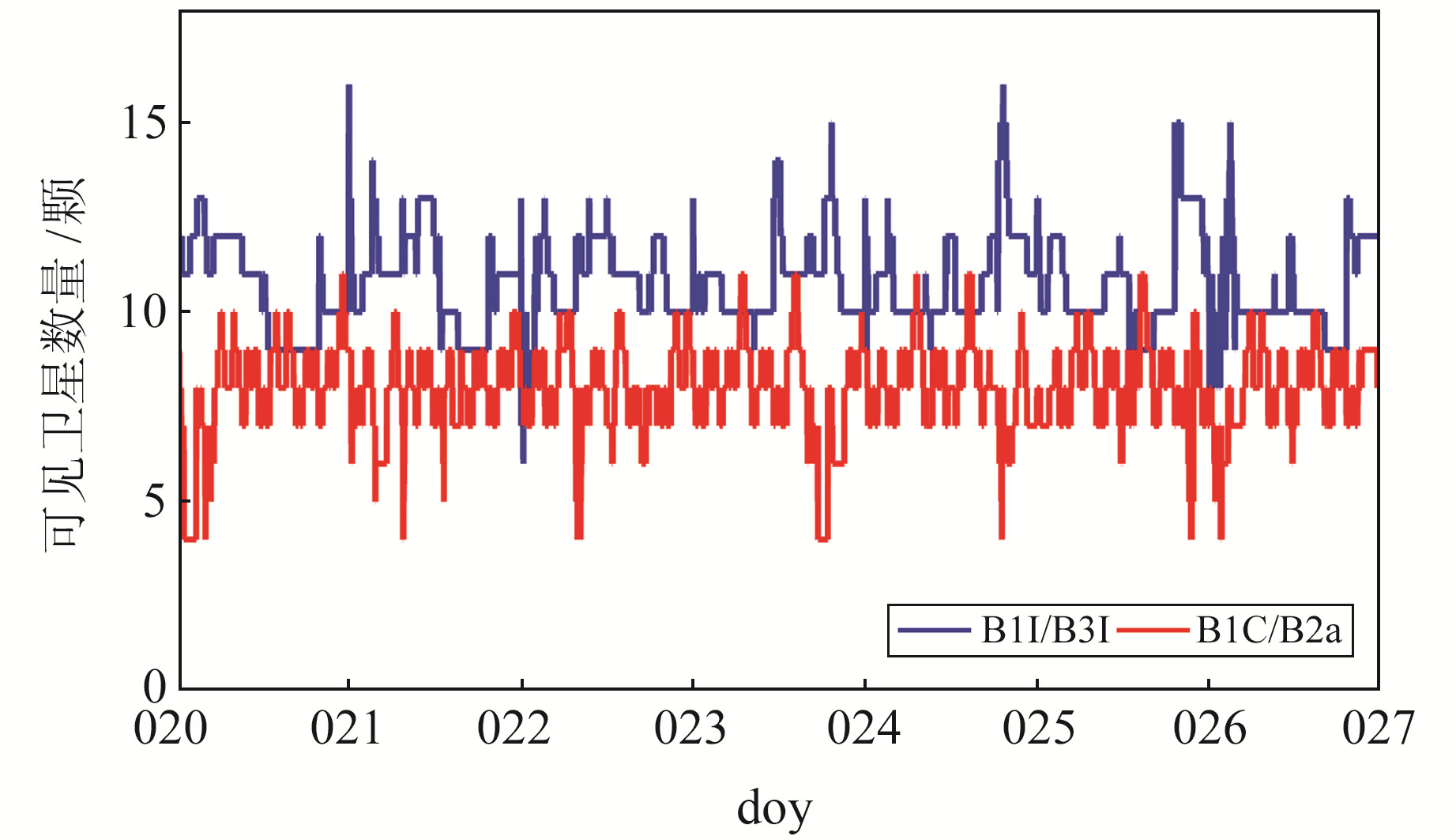图 3 可见卫星数量 Fig. 3 Number of visible satellite
2.1 SPP精度分析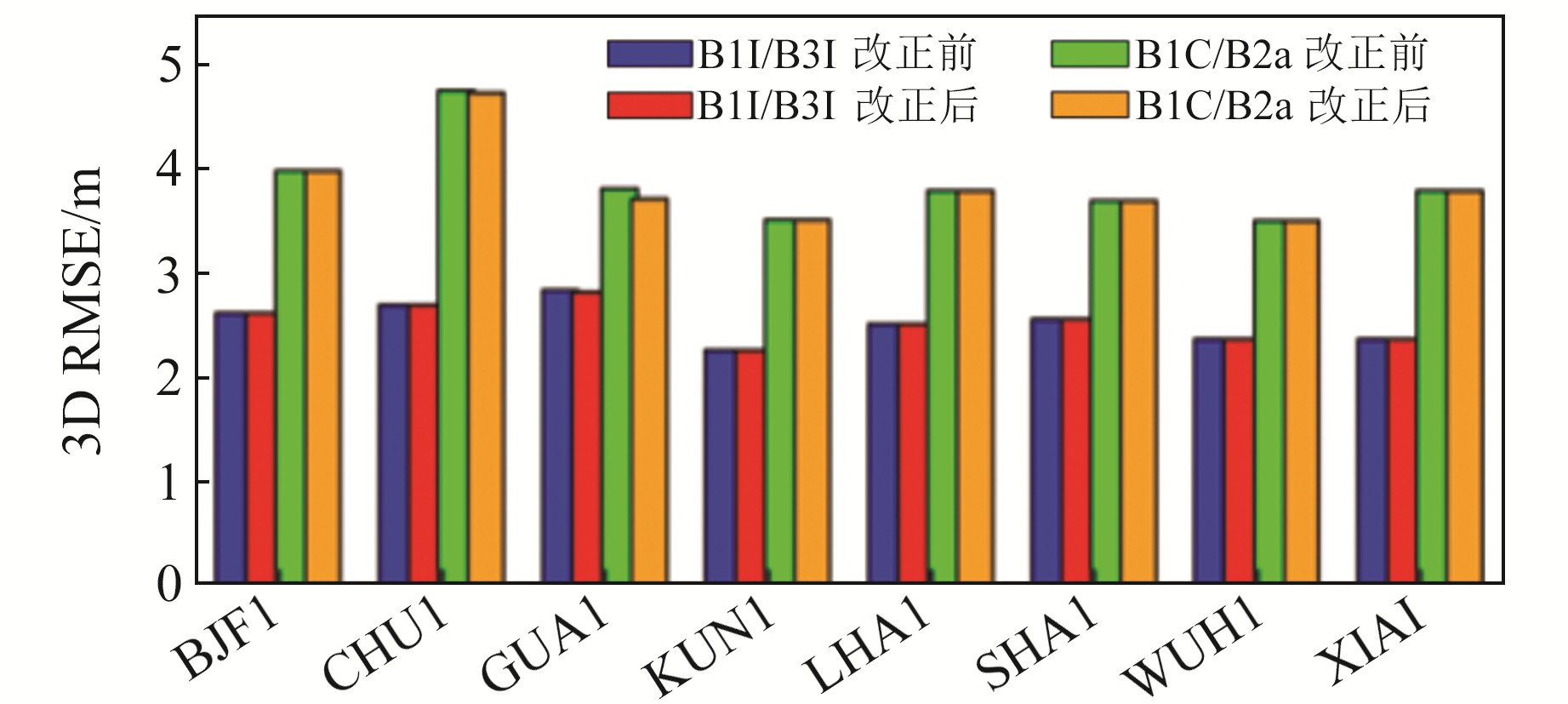图 4 OSB改正前后SPP精度 Fig. 4 SPP accuracy before and after OSB correction
2.2 PPP精度分析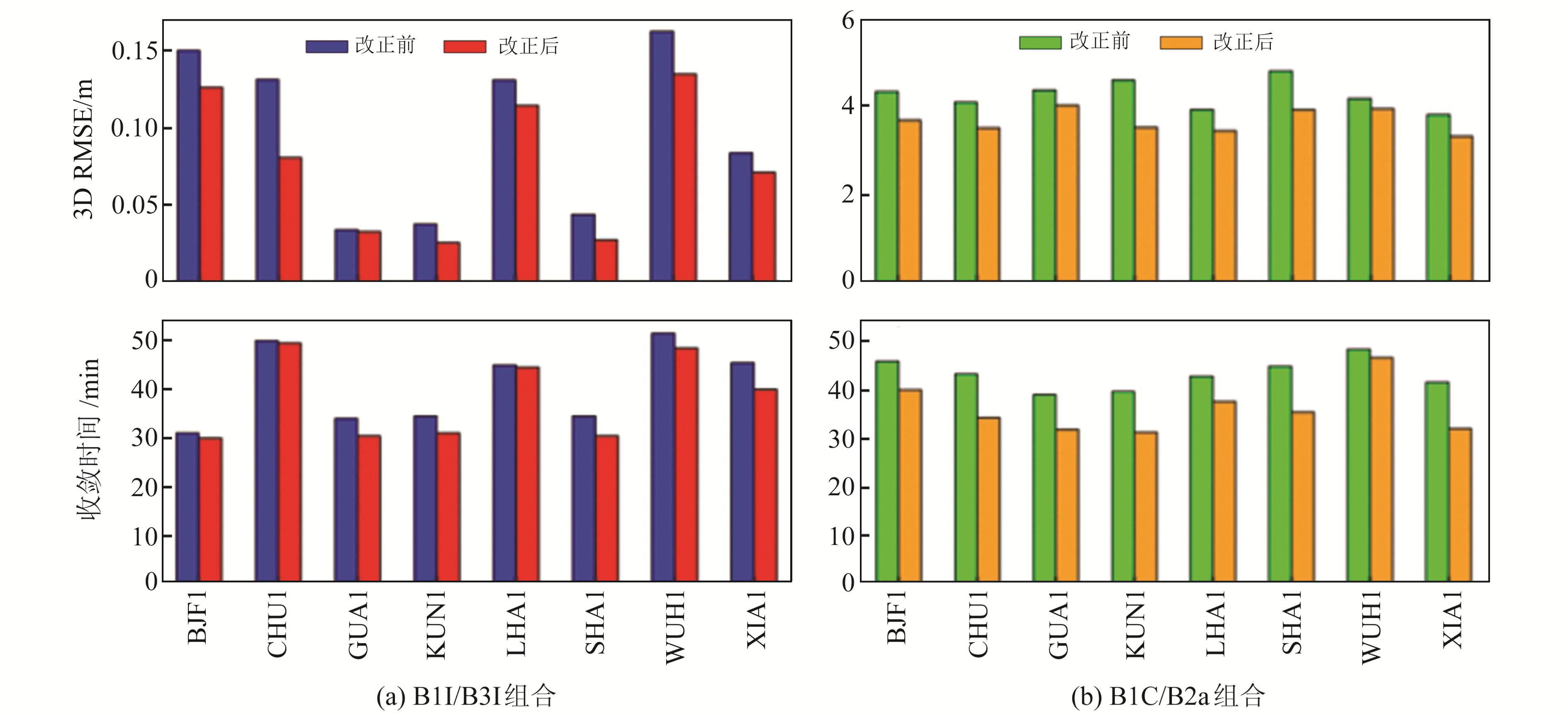图 5 各测站OSB改正前后PPP精度和收敛时间 Fig. 5 PPP accuracy and convergence time before and after OSB correction at each station

3 结语

1) BDS-2/3卫星B1I、B3I、B1C和B2a各频点的OSB估计均值为－80~70 ns，STD精度分别为3.41 ns、5.87 ns、2.04 ns和2.32 ns，BDS不同类型卫星在不同频点上的OSB值也各不相同。综合来看，B1C和B2a频点的稳定性较好。

2) 在SPP方面，OSB改正对B1I/B3I组合和B1C/B2a组合的定位精度影响并不明显。OSB改正后，B1I/B3I组合和B1C/B2a组合的SPP精度分别为2.53 m和3.84 m。

3) 在PPP方面，OSB改正对B1I/B3I组合和B1C/B2a组合的定位精度影响较为明显。对于B1I/B3I组合而言，PPP精度和收敛时间分别提升20.6%和7.3%；B1C/B2a组合的PPP精度和收敛时间分别提升11.9%和16.3%。

  张小红, 左翔, 李盼, 等. BDS/GPS精密单点定位收敛时间与定位精度的比较[J]. 测绘学报, 2015, 44(3): 250-256 (Zhang Xiaohong, Zuo Xiang, Li Pan, et al. Convergence Time and Positioning Accuracy Comparison between BDS and GPS Precise Point Positioning[J]. Acta Geodaetica et Cartographica Sinica, 2015, 44(3): 250-256) (0)  赵文. 北斗2/3联合精密单点定位关键技术研究[D]. 武汉: 武汉大学, 2020 (Zhao Wen. Research on Key Technology of Combined Beidou-2 and Beidou-3 Precise Point Positioning[D]. Wuhan: Wuhan University, 2020) (0)  蔡洪亮, 孟轶男, 耿长江, 等. 北斗三号全球导航卫星系统服务性能评估: 定位导航授时、星基增强、精密单点定位、短报文通信与国际搜救[J]. 测绘学报, 2021, 50(4): 427-435 (Cai Hongliang, Meng Yinan, Geng Changjiang, et al. BDS-3 Performance Assessment: PNT, SBAS, PPP, SMC and SAR[J]. Acta Geodaetica et Cartographica Sinica, 2021, 50(4): 427-435) (0)  Wilson B M, Yinger C, Feess W, et al. New and Improved: The Broadcast Interfrequency Biases[J]. GPS World, 1999, 10(9): 56-66 (0)  Villiger A, Schaer S, Dach R, et al. Determination of GNSS Pseudo-Absolute Code Biases and Their Long-Term Combination[J]. Journal of Geodesy, 2019, 93(9): 1487-1500 DOI:10.1007/s00190-019-01262-w (0)  Deng Y F, Guo F, Ren X D, et al. Estimation and Analysis of Multi-GNSS Observable-Specific Code Biases[J]. GPS Solutions, 2021, 25(3): 1-13 (0)  Paziewski J, Wielgosz P. Accounting for Galileo-GPS Inter-System Biases in Precise Satellite Positioning[J]. Journal of Geodesy, 2015, 89(1): 81-93 DOI:10.1007/s00190-014-0763-3 (0)  Sanz J, Miguel J J, Rovira-Garcia A, et al. GPS Differential Code Biases Determination: Methodology and Analysis[J]. GPS Solutions, 2017, 21(4): 1549-1561 DOI:10.1007/s10291-017-0634-5 (0)  Wanninger L. Carrier-Phase Inter-Frequency Biases of GLONASS Receivers[J]. Journal of Geodesy, 2012, 86(2): 139-148 DOI:10.1007/s00190-011-0502-y (0)  樊家琛, 吴晓莉, 李宇翔, 等. 基于三频数据的北斗卫星导航系统DCB参数精度评估方法[J]. 中国空间科学技术, 2013, 33(4): 62-70 (Fan Jiachen, Wu Xiaoli, Li Yuxiang, et al. COMPASS Satellites DCB Parameter Accuracy Assessment Based on Tri-Frequency Data[J]. Chinese Space Science and Technology, 2013, 33(4): 62-70) (0)  李子申, 王宁波, 袁运斌. 多模多频卫星导航系统码偏差统一定义与处理方法[J]. 导航定位与授时, 2020, 7(5): 10-20 (Li Zishen, Wang Ningbo, Yuan Yunbin. A Unified Definition and Processing Method of Observable-Specific Signal Biases for Multi-Mode and Multi-Frequency Global Navigation Satellite System[J]. Navigation Positioning and Timing, 2020, 7(5): 10-20) (0)  Montenbruck O, Hauschild A, Steigenberger P. Differential Code Bias Estimation Using Multi-GNSS Observations and Global Ionosphere Maps[J]. Navigation, 2014, 61(3): 191-201 (0)  Wang Q S, Jin S G, Yuan L L, et al. Estimation and Analysis of BDS-3 Differential Code Biases from MGEX Observations[J]. Remote Sensing, 2019, 12(1): 68 (0)  Dai P P, Xing J P, Ge Y L, et al. The Effect of BDS-3 Time Group Delay and Differential Code Bias Corrections on Positioning[J]. Applied Sciences, 2020, 11(1): 104 (0)  Wang N B, Li Z S, Duan B B, et al. GPS and GLONASS Observable-Specific Code Bias Estimation: Comparison of Solutions from the IGS and MGEX Networks[J]. Journal of Geodesy, 2020, 94(8): 1-15 (0)
Precision Single Point Positioning Accuracy Analysis of BDS-3 New Frequencies Based on OSB Correction
GU Jiachen1SONG Chuanfeng2TIAN Kunjun3
1. Shandong Wullsion Information Technology Co Ltd, 67 Yubeilou Road, Tai'an 271000, China;
2. GNSS Research Center, Wuhan University, 129 Luoyu Road, Wuhan 430079, China;
3. School of Civil and Architectural Engineering, Shandong University of Technology, 266 West-Xincun Road, Zibo 255000, China
Abstract: Based on the BDS-2/3 satellites observable-specific signal biases(OSB) correction product published by Wuhan University observation data of eight iGMAS stations in China for one month, we analyze the influence of OSB correction on the pseudo-distance single point positioning and precision point positioning accuracy of BDS-2/3 under the combination mode of B1I/B3I old frequency points and B1C/B2a new frequency points. The results show that the mean of B1I, B3I, B1C and B2a OSB ranged from －80 ns to 70 ns, and the annual stability of OSB at each frequency point are 3.41 ns, 5.87 ns, 2.04 ns and 2.32 ns, respectively. In terms of BDS-2/3 pseudo-range single point positioning, after using OSB product correction, the positioning accuracy of B1I/B3I combination is better than 2.53 m, the positioning accuracy of B1C/B2a combination is better than 3.84 m, and the accuracy improvement is not obvious. In terms of BDS-2/3 precise point positioning, the positioning accuracy of 3D direction using B1I/B3I is better than 7.7 cm, the improvement is about 20.6%, the convergence time is about 38 min, the improvement is about 7.3%. The positioning accuracy of 3D direction of B1C/B2a is better than 3.7 cm, and the improvement is about 11.9%. The convergence time is about 36 min, improved about 16.3%.
Key words: BDS-2/3; OSB; DCB; SPP; PPP